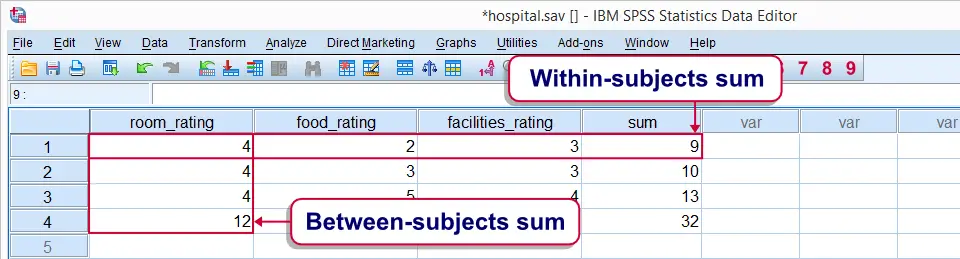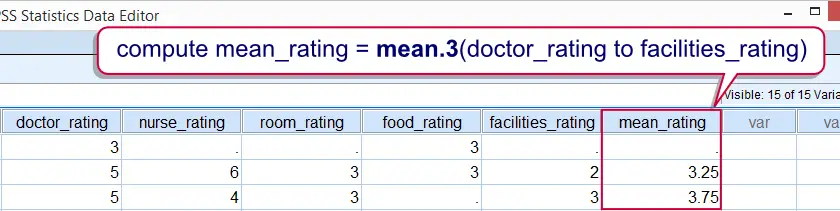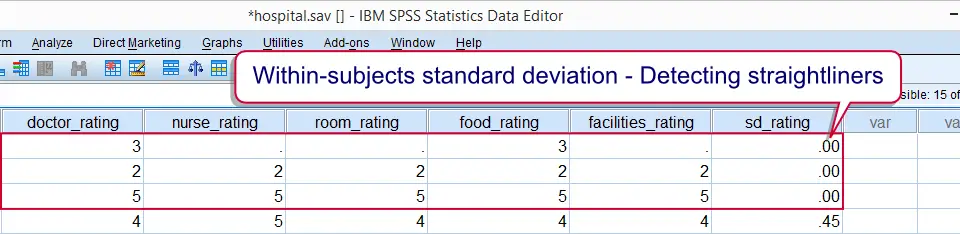SPSS TUTORIALS BASICS ANOVA REGRESSION FACTOR CORRELATION

# SPSS – Quick Overview Statistical Functions

This tutorial walks you through SPSS' main statistical functions. They are mainly used with COMPUTE and IF. Note that these are all within-subjects (or “horizontal”) functions.

For between-subjects (or “vertical”) functions, see AGGREGATE.Within-subjects versus between-subjects functions.

## SPSS Statistical Functions - Missing Values

SPSS statistical functions only return system missing values if all their input values are missing values. If a single input value is valid, the output value will be valid too. This holds for all functions we'll cover in this tutorial.
Remember that the opposite holds for SPSS numeric functions: the latter only return a valid value if all their input values are valid.

## SPSS Statistical Functions - Dot Operator

A minimal number of valid input values can be specified for statistical functions. This is done by suffixing the function with a period followed by the required number of valid values. For example compute mean_v = mean.3(v1 to v5). means “Compute mean_v only for cases having at least 3 valid values over v1 to v5. Cases with fewer valid values must get a system missing value on mean_v.”
The dot operator can be used with all functions covered in this tutorial. Don't overlook it. Although it's little known among SPSS users, it's a terrific time saving feature.Compute mean only for cases with at least 3 valid values on the input variables

## Data Preparation

We'll use only the last 5 variables in our data.Strictly, calculations are not allowed on such ordinal variables. However, see Assumption of Equal Intervals. The functions we'll demonstrate on them may return incorrect values if we fail to specify user missing values. We'll therefore do a quick check by running FREQUENCIES with the syntax below. Note the TO keyword in step 5.

*1. Specify folder where data are located.

cd 'd:/temp'.

*2. Open data file.

get file 'hospital.sav'.

*3. Show values and value labels in output.

set tnumbers both.

*4. Inspect frequencies.

frequencies doctor_rating to facilities_rating.

*5. Specify 6 as user missing value for all variables involved.

missing values doctor_rating to facilities_rating(6).

## SPSS MEAN Function

Means over variables are returned by SPSS MEAN function. If missing values are present, the sum of the valid values is divided by the number of valid values. The syntax below shows how to compute within-subjects means.

*Compute mean_rating as mean over all 5 ratings.

compute mean_rating = mean(doctor_rating to facilities_rating).
exe.

## SPSS SUM Function

SPSS SUM function returns the sum over a number of variables. In the presence of missing values, the sum over all valid values is returned. Keep in mind that the result may be somewhat misleading in this case.Also see SPSS Sum - Cautionary Note. The syntax below computes the within-subjects sum over our rating variables.

*Compute sum over 5 ratings.

compute sum_rating = sum(doctor_rating to facilities_rating).
exe.

## SPSS MIN Function

The minimum (smallest value) over a number of values is returned by SPSS MIN function. We normally use MIN for numeric variables but it can technically be used on string variables as well. It's demonstrated on our rating variables by the syntax below.

*Compute minimum value over variables.

compute min_rating = min(doctor_rating to facilities_rating).
exe.

## SPSS MAX Function

SPSS MAX function returns the maximum (largest value) over a number of values. Just like MIN, it can be used on string variables too. The syntax below computes the maximum over the rating variables.

*Compute maximum rating.

compute max_rating = max(doctor_rating to facilities_rating).
exe.

## SPSS SD Function

The standard deviation over a number of variables is returned by SPSS SD function. Keep in mind that we're referring to the within-subjects standard deviation here.SPSS divides by (n-1) when computing the standard deviation.
Computing within-subjects standard deviations comes in handy in survey research for detecting straightliners: respondents who give the same answer to all questions will have a standard deviation of zero over these questions. This may be an indication that the questions weren't answered seriously, in which case you may want to exclude such cases from analysis.See SELECT IF and FILTER for the most likely options here.

## SPSS SD Function Syntax Example

*1. Compute within-subjects standard deviation over rating variables.

compute sd_rating = sd(doctor_rating to facilities_rating).
exe.

*2. Move straightliners to top of file.

sort cases by sd_rating.

*3. Delete straightliners from data.

select if sd_rating > 0.
exe.Detecting potential straightliners with SPSS SD function.

## SPSS VARIANCE Function

SPSS VARIANCE function computes the within-subjects variance over a number of variables. It's simply the squared standard deviation.

*Compute within-subjects variance over rating variables.

compute variance_rating = variance(doctor_rating to facilities_rating).
exe.

## SPSS MEDIAN Function

Finally, the median over a number of values is returned by SPSS MEDIAN function. Again, note that we refer to the within-subjects median. The syntax below demonstrates it on our rating variables.

*Compute within-subjects median over rating variables.

compute median_rating = median(doctor_rating to facilities_rating).
exe.

# Tell us what you think!

*Required field. Your comment will show up after approval from a moderator.

# THIS TUTORIAL HAS 16 COMMENTS:

•### By Ruben Geert van den Berg on March 1st, 2017

Hi Eline!

I'd first make sure you have 5 variables representing the 5 flavors. Then compute the absolute differences between those and 50 as 5 new variables. Then find which of those 5 variables holds the smallest value for each participant.

I didn't entirely get the second part of the question. I could take a look at your data and fix it for you but I'd have to charge 1 or 2 hours for it.